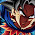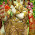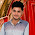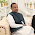Monday, 25 March 2013

CBSE Class 10 - CH 12 - Electricity (MCQs)

ElectricityAn electric circuit

MCQs

Q1: The SI unit of electric charge is __________

(a) volts (V)
(b) ampere (A)
(c) coulomb (C)
(d) tesla (T)

Q2: By convention, the charge on a glass rod that has been rubbed with silk is called

(a) Positive
(b) Negative
(c) either positive or negative
(d) none of these

Q3: Two charged bodies having equal potential are connected through a conducting wire, in this case

(a) current will flow
(b) current will not flow
(c) cannot say
(d) current will flow if a resistor is also connected.

Q4: The Potential Difference between two terminals can be measured by _________

(a) an ammeter.
(b) a voltmeter
(c) an ohm-meter
(d) a rheostat

Q5: The net charge flowing through a cross section of a conductor in unit time is known as

(a) ampere
(b) volt
(c) resistance
(d) electric potential

Q6: Which of the following correctly represents the relation among Charge (Q), Potential difference (V) and  Work done (W)

(a) V = W × Q
(b) W = V / Q
(c) V = Q / W
(d) V = W / Q

Q7: The voltmeter is always connected in _________ across the points between which the potential difference is to be measured.

(a) series
(b) parallel
(c) either series or parallel
(d) none of these

Q8: How many electrons will be there in 1.6C charge?

(a) 1017
(b) 1018
(c) 1019
(d) 1020

Q9: According to Ohm's law,

(a) The resistance increases with the increases in current.
(b) The resistance increases with the increases in voltage.
(c) The current increases with the increases in voltage.
(d) The resistance and current both increases with the increases in voltage.

Q10: A voltmeter is used to measure
(a) potential difference
(b) electric current
(c) electric power
(d) resistance

Q11: On which of the following no “plus” or “minus” sign is marked
(a) a battery
(b) an ammeter
(c) a voltameter
(d) a resistor

Q12: A galvanometer is used to

(a) measure potential difference
(b) detect direction of current
(c) measure amount of current flowing
(d) measure electric power of the circuit

Q13: An electric fuse is based on
(a) the heating effect of the current
(b) the chemical effect of the current
(c) the magnetic effect of the current
(d) none of these

1: (c) coulomb (C)
2: (a) Positive
3: (b) current will not flow
4: (b) a voltmeter
5: (a) ampere
6: (d) V = W / Q
7: (b) parallel
8: (c) 1019 [Hint 1C = 6.25 × 1018electrons ⇒ 1.6C will have  = 1.6 × 6.25 × 1018]
9: (c) The current increases with the increases in voltage.
10: (a) potential difference
11: (d) a resistor
12: (b) detect direction of current
13: (a) the heating effect of the current1.this was really helpful.thanks a lot......

2.THIS WAS NOT RELATED TO ALL THE INFORMATION

1.2.Some are right pandu

3.Why do you think so??

4.5.its awesome.HANDS OFF TO U GUYS :-)

6.7.This comment has been removed by the author.

8.nice site to browse...

9.nice site to browse...

10.nice... but 2 zimple..

11.should include board question exspecially numericalzzz...

1.yep we need some of that too.

12.Its Awsm but simple

13.Informative ND nccc

14.A current of 0.6 A is shown by ammeter in the circuit when the key K1 is closed. Find the resistance of the lamp L. What change in current flowing through the 5 Ω resistor and potential difference across the lamp will take place, if the K2 key is also closed? Give reason for your answer.

15.16.17.18.